# CQG Primer: Oscillators

Oscillators are very popular studies used by trader and analysts alike. Oscillators detrend the price data by calculating differences. The next image displays a simple oscillator named Momentum. It is the close - close 14 days ago. If the closing price is higher than the close 14 days ago then the oscillator is positive and if the close is below the close 14 days ago then the oscillator is negative. This particular oscillator is not bounded. Therefore the oscillator values can be any value. Some oscillators are bounded; that is the values cannot be less than zero or greater than 100.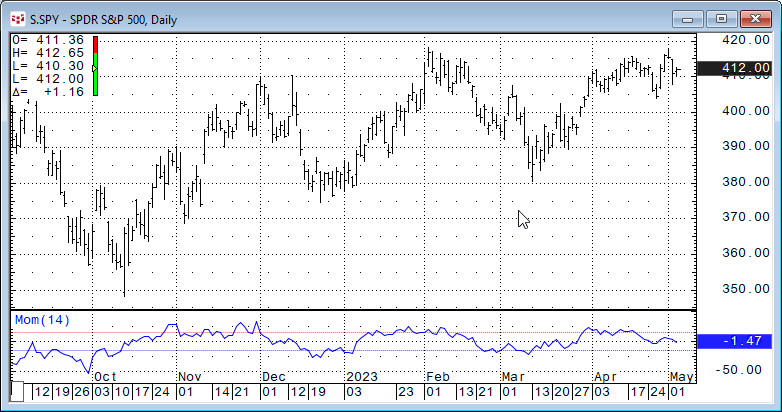One example of how traders and analysts look at oscillators is they conclude that if an oscillator is not generating higher values while the market is making new highs then that divergence indicates a loss of upside momentum and a reversal may be in the offering. Similarly, if the market is making new lows and the oscillator is not displaying lower readings then the downside momentum is abating and a rally may be forthcoming.

There are numerous oscillator based studies available and this article offers an overview and some correlation analysis to gauge how similar many oscillators are to each other. For the correlation comparison the base oscillator will be the simple "close minus the close 14-days ago" which is referred to as the Momentum study. All oscillator studies will use the 14-day lookback period. The range of data is 220 daily bars for the symbol "SPY."

To better understand the correlation analysis of the relationship of the individual oscillators compared to the Momentum oscillator we will use Excel's Frequency function. This function returns the number of times a score lands in a range of values. The frequency function counts the number of times the historical individual correlation value lands in a "bin." The bins range from 1.00 to -1.00 in 0.025 steps.

This approach is gauged towards recognizing that multiple studies used in a trading system may be simply reflecting the same information not confirming the same information.

The name of each oscillator is linked to the respective CQG Help file.

#### Aroon Oscillator

The Aroon Oscillator is the difference the Aroon_Up study and the Aroon_Down study. Aroon_Up and Aroon_Down measure the relative placement of the highest high or lowest low over a given period measured as a percentage from 0 to 100. An uptrend would be values from the Aroon Oscillator over 50, a downtrend would be values less than -50, and a consolidation would be between those two marks.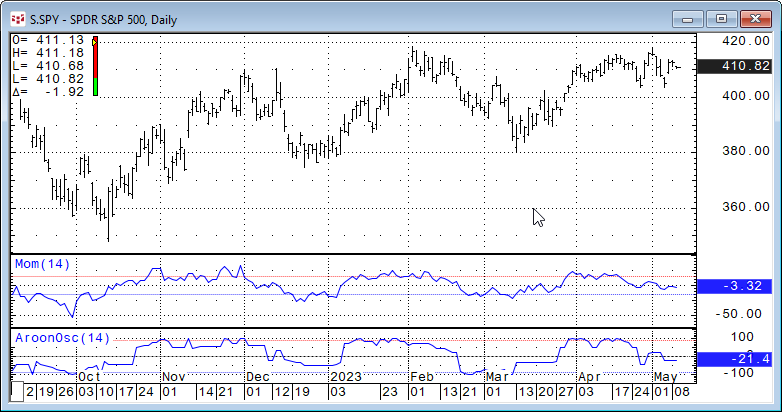A simple visual comparison of the two studies above indicates that the two studies do have a tendency to have similar outputs.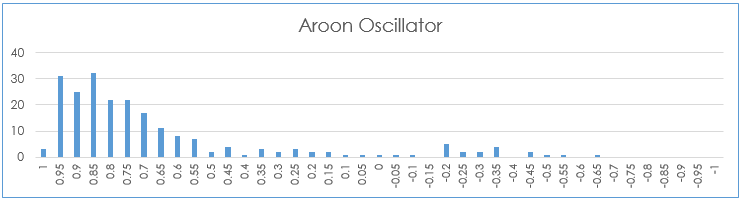The frequency chart indicates the level the two oscillators track each other with 132 of the 220 observations or 60% of the observations 0.75 or higher. There were 20 observations below 0.

#### Commodity Channel Index

The Commodity Channel Index (CCI) measures the variation of the current price from its statistical mean. The CCI is an oscillator centered around zero.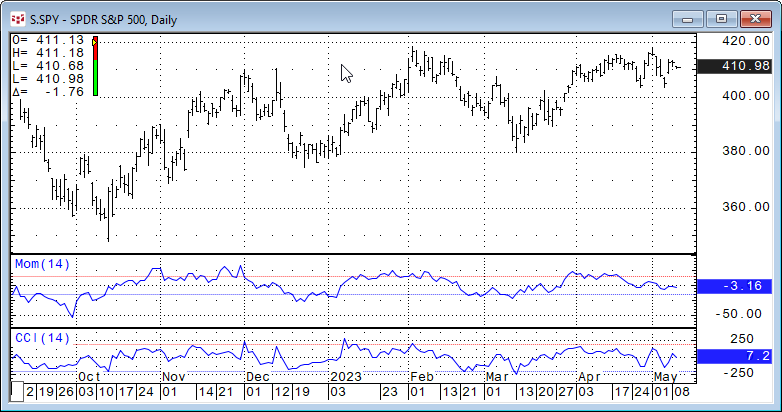The simple visual comparison of the two studies above indicates less correlation between the two studies compared to the Aroon Oscillator versus the Momentum Oscillator.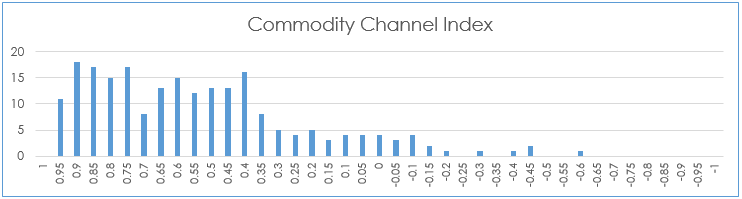The frequency analysis confirms this. There were no correlations values greater than 0.95, only 78 or 35.45% of the 220 observations were greater than 0.75.

#### Directional Movement Index Difference

The DMI Difference is a derivative of the DMI study. The calculation subtracts the DMId from the DMIu line. Positive numbers indicate an uptrend and negative numbers indicate a downtrend. Numbers oscillating around zero indicate the absence of a trend.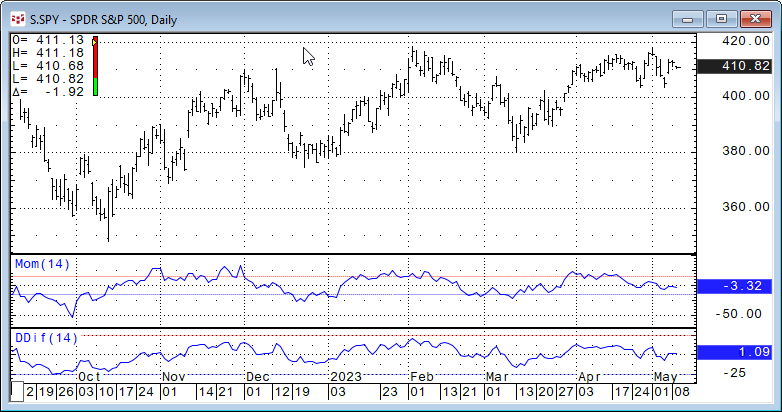These two oscillators above look fairly coincident.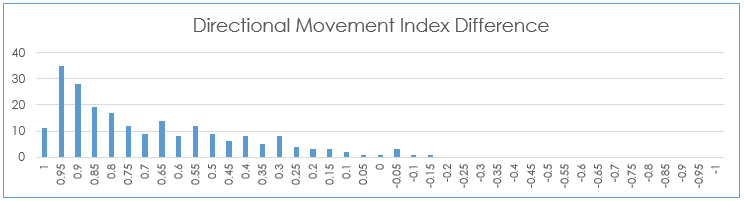The Frequency analysis does show that 11 times the correlation was greater than 0.95, 111 or 50.45% of the 220 observations were greater than 0.75.

#### Fast Stochastics

Fast %K - The system identifies the highest high, lowest low, and the current price for a specified period. The formula is:

`%K= ((Close(@)- LoLevel(@,14))/ (HiLevel(@,14)- LoLevel(@,14)))*100`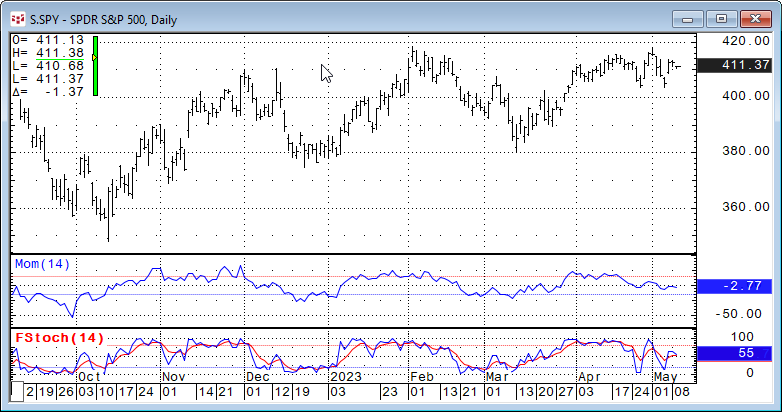This study produces two plots: %K and %D. %D is smoothed version of %K.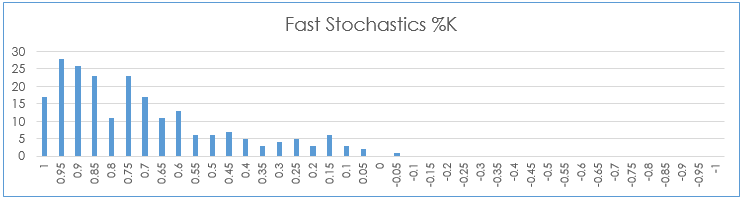The Frequency analysis shows that 17 times the correlation was greater than 0.95, 111 or 50.45% of the 220 observations were greater than 0.75.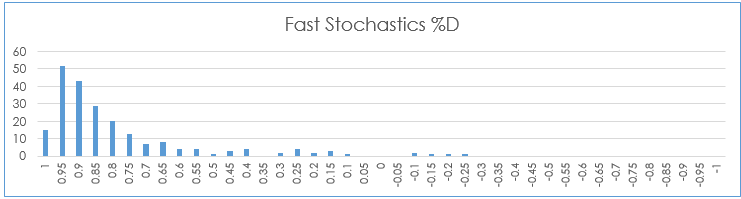The Frequency analysis for the %D line revealed that 157 or 71.36% of the 220 observations was a correlation higher than 0.75. Fifteen times the correlation was greater than 0.95

The Intraday Momentum Index combines aspects of candlestick analysis with the relative strength index (RSI) calculation in order to generate overbought or oversold signals.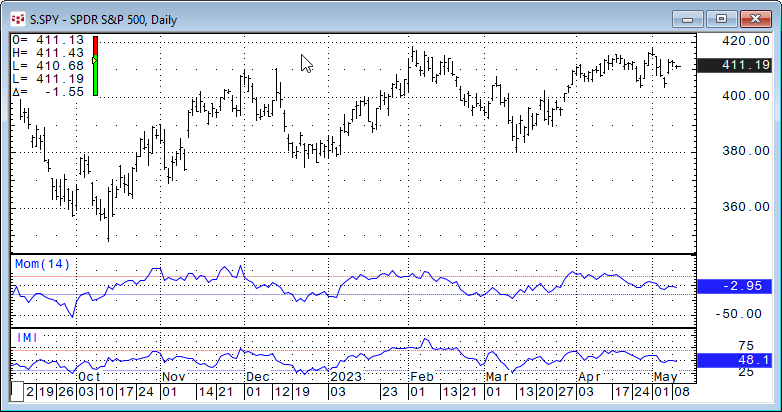A visual inspection indicates the two studies appear to be coincident.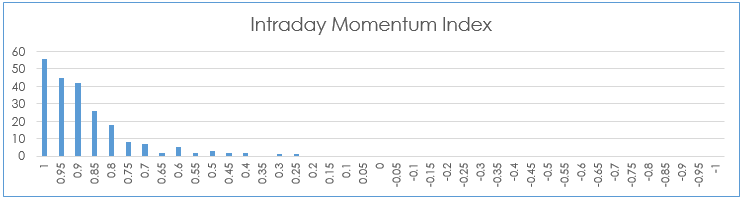The Frequency analysis indicates that 139 or 63.18% of the 220 observations were greater than 0.75. There were 56 times the correlation was greater than 0.95.

#### Moving Average Convergence/Divergence

The MACD measures the difference between two exponential moving averages of different lengths, in addition, a trailing moving average of the MACD is plotted (MACDA), this is commonly referred to as the "Trigger" line.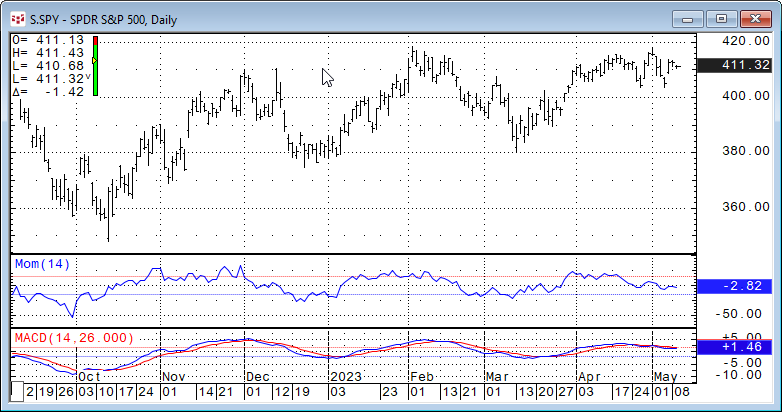Due to the fact that the MACD is the difference between two exponential averages the line is smoother than the Momentum study.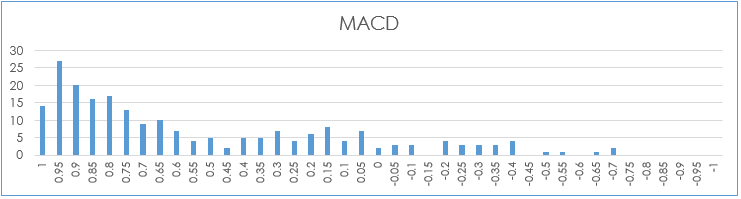The Frequency analysis indicates that 93 or 42.27% of the 220 observations were greater than 0.75. There were 14 times the correlation was greater than 0.95.

#### Oscillator

Similar to the MACD the Oscillator study plots the difference between two moving averages of different lengths or types.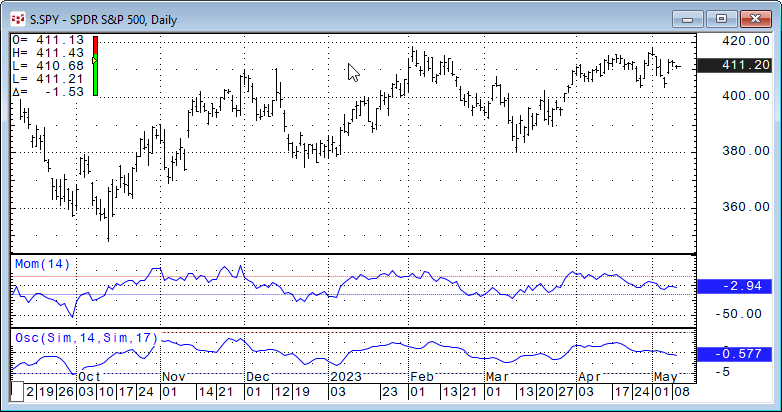Here, the two appear to rise and fall approximately together.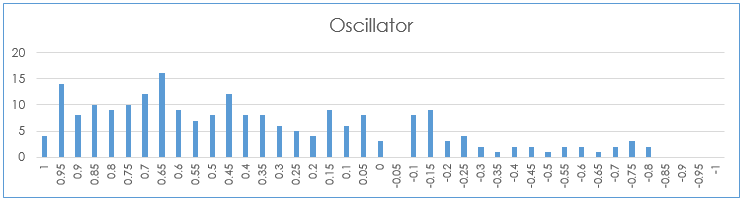The Frequency analysis is widely dispersed. Fifty-one or 23.18 % of the 220 observations were greater than 0.75. There were 14 times the correlation was greater than 0.95.

#### Rate of Change

The Rate of Change study is interesting compared to the Momentum study. The formula for the ROC study is:

`ROC = ((Current Price / Prior Price) * 100) - 100`

Please recall that the Momentum study is Current Price minus Previous price from, in this case, 14 bars ago.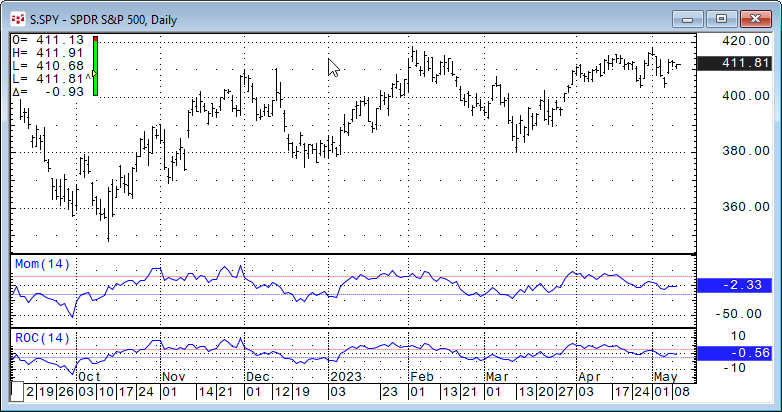The two curves are identical except for the vertical scale. The correlation is +1 for the entire 220 observations.

#### Relative Strength Index

Relative Strength Index is a price momentum indicator. The formula is:

`RSI = 100 - [100/(1+RS)]`

where, RS is the ratio of the smoothed moving average of n-period gains divided by the absolute value of the smoothed moving average of n-period losses.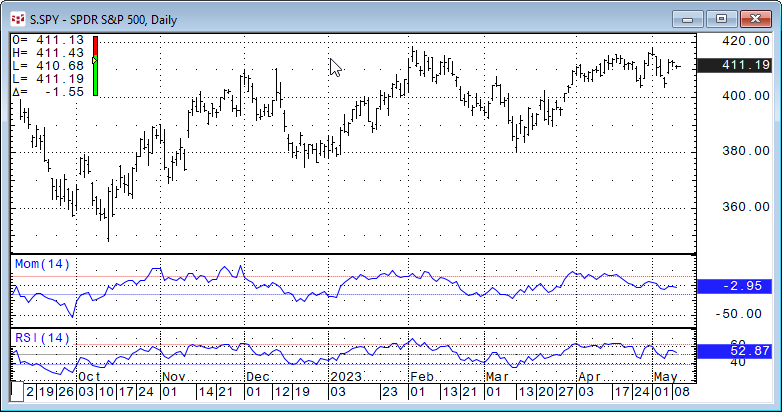The name Relative Strength Index is a misnomer. There is nothing that is "relative" regarding the outputs of this study.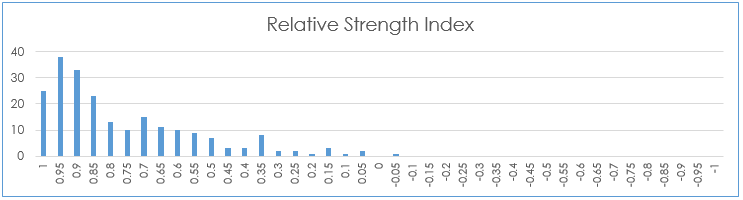The Frequency analysis indicates that 117 or 53.18% of the 220 observations were greater than 0.75. There were 25 times the correlation was greater than 0.95.

#### Slow Stochastics

The Slow Stochastic first calculates the Fast Stochastic. The Slow %K and Slow %D lines are displayed on the screen. Slow %K - Is equal to the Fast %D. Slow %D - Is a moving average of Slow %K values. The default for Slow %D is a Smoothed 3 Period Moving Average.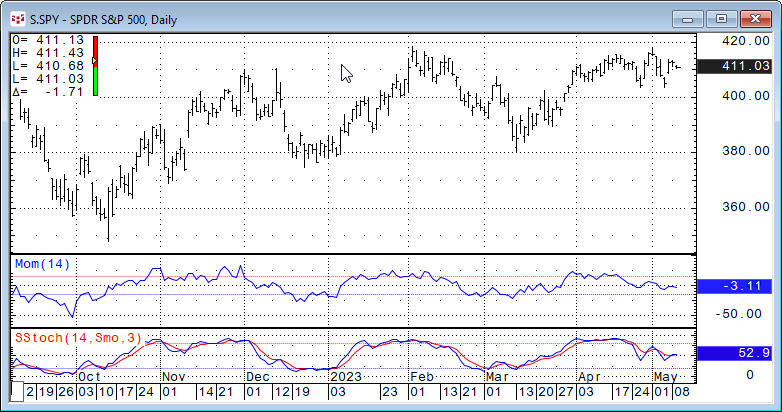Both the Slow %K and Slow %D are displayed.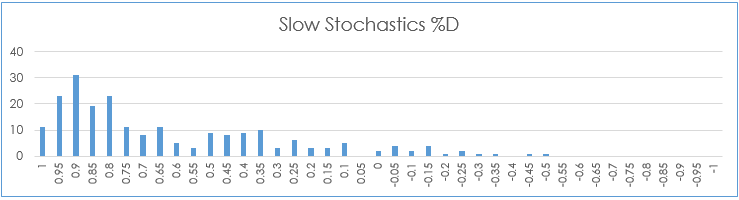The Frequency analysis indicates that 107 or 48.64% of the 220 observations were greater than 0.75. There were 11 times the correlation was greater than 0.95.

#### Summary

The point of this article was to one, offer some insight into the basic calculations and to illustrate that a number of these oscillators are providing duplicate information. One aid

Next, if you are interested in further analysis of the various oscillators for a trading system then studying the writings of CQG's Product Specialist Helmut Mueller posts on backtesting and much more is an excellent place to start.